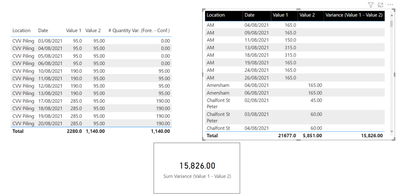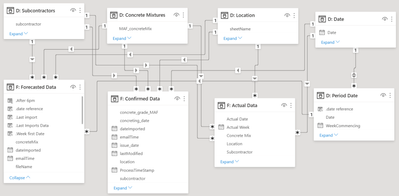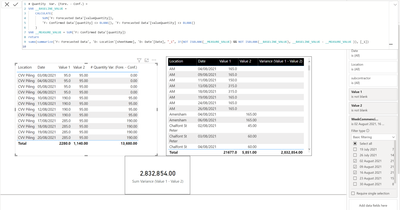cancel
Showing results for
Did you mean:New Member

## Subtracting 2 different columns from 2 different tables

Hi,

I am trying to calculate the difference between 2 values from 2 different tables. The data model consists of 3 tables and 5 dimension tables. The 2 tables that have the columns does not have a direct relationship between them. I want the subtraction to take place only when there is a value in the 2 columns. Also, I want to have the total value of this measure.

The following screenshot presents the tables and the current measures that I managed to produce (not functioning well!!). I want the card to show 1140. It is showing the same number as the value in the second table which is the sum of the values of 'Value 1' in that table minus the sum of values in the 'Value 2'.Here is the dax for the measure on the card:

Variance (Value 1 - Value 2)=
VAR __BASELINE_VALUE =
CALCULATE(
SUM('F: Forecasted Data'[Value 1]),
'F: Confirmed Data'[Value 2] <> BLANK(), 'F: Forecasted Data'[Value 1] <> BLANK()
)
VAR __MEASURE_VALUE = SUM('F: Confirmed Data'[Value 2])
RETURN
IF(NOT ISBLANK(__MEASURE_VALUE) && NOT ISBLANK(__BASELINE_VALUE), __BASELINE_VALUE - __MEASURE_VALUE )

Here is the data model:Thanks
1 ACCEPTED SOLUTIONCommunity Support

Hope the following formula could work.

``````Variance (Value 1 - Value 2) =
VAR tab =
CROSSJOIN ( 'D:Location', 'D:Date' )
VAR tab2 =
FILTER (
tab,
"v1",
CALCULATE (
SUM ( 'F: Forecasted Data'[Value 1] ),
FILTER (
tab,
[Date] = EARLIER ( 'D:Date'[Date] )
&& [sheetName] = EARLIER ( 'D:Location'[sheetName] )
)
),
"v2",
CALCULATE (
SUM ( 'F: Confirmed Data'[Value 2] ),
FILTER (
tab,
[Date] = EARLIER ( 'D:Date'[Date] )
&& [sheetName] = EARLIER ( 'D:Location'[sheetName] )
)
)
),
[v1] <> BLANK ()
&& [v2] <> BLANK ()
)
RETURN
SUMX ( tab2, [v1] - [v2] )
``````

Best Regards,
Community Support Team _ Eason

3 REPLIES 3Super User

@farhanishango , Try return like

return
sumx(summarize('F: Forecasted Data', 'D: Location' [location], Date[Date], "_1", IF(NOT ISBLANK(__MEASURE_VALUE) && NOT ISBLANK(__BASELINE_VALUE), __BASELINE_VALUE - __MEASURE_VALUE )), [_1])New Member

Hi @amitchandak,

Here is what I got:Community Support

Hope the following formula could work.

``````Variance (Value 1 - Value 2) =
VAR tab =
CROSSJOIN ( 'D:Location', 'D:Date' )
VAR tab2 =
FILTER (
tab,
"v1",
CALCULATE (
SUM ( 'F: Forecasted Data'[Value 1] ),
FILTER (
tab,
[Date] = EARLIER ( 'D:Date'[Date] )
&& [sheetName] = EARLIER ( 'D:Location'[sheetName] )
)
),
"v2",
CALCULATE (
SUM ( 'F: Confirmed Data'[Value 2] ),
FILTER (
tab,
[Date] = EARLIER ( 'D:Date'[Date] )
&& [sheetName] = EARLIER ( 'D:Location'[sheetName] )
)
)
),
[v1] <> BLANK ()
&& [v2] <> BLANK ()
)
RETURN
SUMX ( tab2, [v1] - [v2] )
``````

Best Regards,
Community Support Team _ EasonAnnouncements#### Exclusive opportunity for Women!

Join us for a free, hands-on Microsoft workshop led by women trainers for women where you will learn how to build a Dashboard in a Day!#### Power Platform Conference-Power BI and Fabric Sessions

Join us Oct 1 - 6 in Las Vegas for the Microsoft Power Platform Conference.Top Solution Authors
Top Kudoed Authors
Users online (1,682)